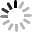News
Home > news > Company news about Accurately Test The Insertion Loss Of RF Cable
Events
Contact Now

# Accurately Test The Insertion Loss Of RF Cable

2019-09-301. Test with TDR method, real-time domain reflection.

2. Time-Domain Reflectometry (TDR) technology. The technology includes the generation of time step voltages propagating along transmission lines. The reflection from impedance is detected by oscilloscope, and the ratio of input voltage to reflection voltage is measured to calculate discontinuous impedance.

3. Data measured by network analyzer in frequency domain can be used to calculate and display network step and impulse response as time function. The traditional TDR capability in reflection and transmission increases the potential for measurement in band-limited networks.

In reflection mode, the network analyzer measures the reflection coefficient as a frequency function. The reflection coefficient can be regarded as a transfer function of the incident voltage and the reflected voltage. The inverse transform transforms the reflection coefficient into a time function (impulse response). The step and impulse responses can be calculated by convolution of the reflection coefficient with the input step or pulse. In transmission mode. The network analyzer measures the transfer function of a two-port device as a frequency function. The inverse transformation transforms the transfer function into the impulse response of a two-port device. The step and impulse responses are calculated by convolution of the impulse response with the input step or pulse.

Send your inquiry directly to us